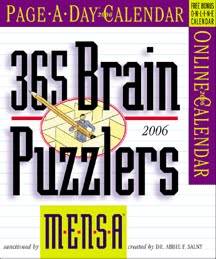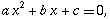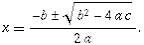## Monday, August 07, 2006

### Mensa Page-A-Day PuzzleI had gotten the Page-a-day Mensa Puzzle Calendar as a gift from a real Mensa member (I am too cheap to join such organizations myself). Usually, I buzz through it quickly every day, but for some reason, I had gotten stuck on this one from May 10th. It sat on my desk at work until just last week when I finally solved it. Perhaps I simply didn't have enough room on the calendar page itself to solve it, or I had too much work to do to spend more than 5 min, but more likely it was a fear that I had to perform quadratic equations or something. Speaking of quadratic equations, this funny segment from APM's Marketplace about comes to mind. Just listening to it made me cringe as I couldn't remember for the life of me what it was, only that it had ± and 4ac in it. Here it is to remind all of you who have forgotten:
forIn all my work experience, I hadn't even thought about the quadratic equation since high school. Is it really a necessary evil? Anyways, back to that pesky Mensa problem (hint, no quadratic equations are needed to solve it):
Eric is driving his car to the airport to pick up his wife. At his current speed, he'll arrive on time. If he drives 5 miles per hour faster, he'll arrive 12 minutes early, but if he drives 5 miles per hour slower, he'll arrive 15 mintues late. How far away is the airport?

There was another Mensa puzzle which I found had an error in it!

Kim has three vases in her living room, each containing the same number of flowers. Kim adds three fresh flowers to one vase, which now has two more than the new average. How many flowers were in each vase originally?
I gave this one to my friend, Jennifer, after giving her some post-op flowers and her response was:
yes - algebra while I'm recovering?

gee thanks Angela

And of course, Dave, the person who gave me the calendar, replied in all of 6 minutes.
X + 3 = 2 + (3X + 3)/3

X + 1 = X + 1

Any initial number of flowers will work.

Or, if you don't like algebra.
Adding 3 flowers will increase the average by 1, so the new vase will
always have 2 more than the average.
Those puzzle editors are a bunch of dopes! There was another minor error just last Saturday which they did fess up to on their website. We'll see what they say about the previous error.
technorati tags:,Anonymous said...

I cheated a little.....but here it is anyways:

1) Distance is equal in all three cases.
- Case 1: Base speed and time
- Case 2: Base speed+5, time-12min
- Case 3: Base speed-5, time+15min
2) Use MPH as base units, convert to same units: 12 minutes = 0.2 hrs, 15 minutes = 0.25 hrs
3) From the cases:
D = (s+5)*(t-0.2) = (s-5)*(t+0.25)
4) Solve for T: 10t=0.45s-0.25
t=0.045s-0.025
5) Here is where I cheated and used a spreadsheet. The concept is that the three sets of data given must come out to the same distance....so I just took the equation and solved with the three sets of data.

Where the D (distance) intersected....the answer.

a) I made my first column called with
values of speed (s) from 0 to 200.
b) my 2nd column calculated "t"
from the above equation.
c) my third column calculated Distance
as "speed x time"
(here is where my mind gets convoluted)
d) the next column takes column "a"
and adds 5 (for first case with
+5mph) I call this S1 for speed 1
e) next column take column "b" and
subtracts 12/60 (12 minutes in hrs)
I call this t1, for the time case 1
f) next column calculates D1, or the
distance of case #1 by multiplying
s1 by t1
g) the next column takes column "a"
and subtracts 5 (for first case
with -5mph) I call this S2 for
speed of case 2
h) next column take column "b" and
add 15/60 (15 minutes in hrs)
I call this t2, for the time case 2
i) next column calculates D2, or the
distance of case #2 by multiplying
s2 by t2

Again, the answer is in the row where D=D1=D2

This happens at D=90 (miles)

I will email you the spreadsheet to prove my insanity. (i'll even scan my notepad and email it if that helps prove it :-)

Angela said...

Spreadsheet? I guess that would be the empirical way to do it.. It was only algebra for crying out loud, and the solution fits easily onto a 1/2 sheet of regular paper.

I'll be out at a seminar today, so I'll work on your "prize" tonight!

Unknown said...

Not as straightforward as I thought, but here goes.

Three equations:
1) d=rt
2) d=(r+5)(d-12/60)
3) d=(r-5)(d+15/60

Combine 1) and 2)
rt=(r+5)(d-12/60)
Math consolidates to 5t=12r/60+1

Combine 1) and 3)
rt=(r-5)(d+15/60)
Math consolidates to 5t=15r/60-75/60

5t=5t, so 12r/60+1=15r/60-75/60
Math consolidates to r=45

Plug r=45 back into previous result of 5t=12r/60+1 or 5t=15r/60-75/60 and math consolidates to t=2

Plug back into equation 1) and d = 90